不写R包的分析师不是好全栈

# 将聚类聚类

R

• 基于连通性的聚类: 层次聚类
• 基于中心的聚类: kmeans聚类
• 基于分布的聚类: GMM聚类
• 基于密度的聚类: DBSCAN,OPTICS
• 基于模型的聚类: Miture Regression Model
• 其他聚类方法: 谱聚类,Chameleon,Canopy…

## 基于连通性的聚类

基于连通性聚类,也叫做层次聚类(hierarchical clustering).这种聚类的思路是:每个观测值离它较近观测值更可能是一类,而不是离它更远的观测值.基本的算法是使用分步算法,每次将离得最近的两个点(两族点)合并成新的一族点,经过n-1步的合并,得到最终的包含所有点的一个类,过程可以画出树状图,依据自己想要的类的个数进行选择.

Algorithm:

1. 计算距离矩阵(用到定义的距离和连接准则)

2. 找到距离最小的两个点,合并为一个更大的点(一族点)

重复1-2步直到合并为一个包含所有数据的点

Metric:

namesEng.formula
欧氏距离Euclidean distance(||a-b||_2 = \sqrt{\sum_i (a_i-b_i)^2})
平方欧氏距离Squared Euclidean distance(||a-b||_2^2 = \sum_i (a_i-b_i)^2)
马氏距离Manhattan distance(||a-b||_1 = \sum_i |a_i-b_i|)
极大值距离Maximum distance(||a-b||{\infty} = {max}{i} |a_i-b_i|)
统计距离Mahalanobis distance(\sqrt{(a-b)\top S{-1}(a-b)}) (S) 是样本协差阵

NamesEng.Formula
极大距离Maximum or complete linkage clustering(\max \, {\, d(a,b) : a \in A,\, b \in B \,})
极小值距离Minimum or single-linkage clustering(\min \, {\, d(a,b) : a \in A,\, b \in B \,})
平均距离Mean or average linkage clustering, or UPGMA(\frac{1}{|A| |B|} \sum_{a \in A }\sum_{ b \in B} d(a,b))
中心距离Centroid linkage clustering, or UPGMC(||c_s - c_t ||) (c_s)(c_t)(s)(t)的中心.
能量聚类Minimum energy clustering(\frac {2}{nm}\sum_{i,j=1}^{n,m} |a_i- b_j|2 - \frac {1}{n^2}\sum{i,j=1}^{n} |a_i-a_j|2) (- \frac{1}{m^2}\sum{i,j=1}^{m} |b_i-b_j|2)

### Example

dist函数默认使用欧式距离进行聚类,类似其他的距离定义可以用method来定义:

• method

• the distance measure to be used. This must be one of "euclidean", "maximum", "manhattan", "canberra", "binary" or "minkowski". Any unambiguous substring can be given.

hclust函数对数据进行聚类,连接准则使用“ave”

• method

• the agglomeration method to be used. This should be (an unambiguous abbreviation of) one of "ward.D", "ward.D2", "single", "complete", "average" (= UPGMA), "mcquitty" (= WPGMA), "median" (= WPGMC) or "centroid" (= UPGMC).

dist(c(1:5))

##   1 2 3 4## 2 1## 3 2 1## 4 3 2 1## 5 4 3 2 1

## default:method = "euclidean"## Show the distance of the vetorhc <- hclust(dist(USArrests), "ave")## hc is the answer of hierarchical clusteringplot(hc)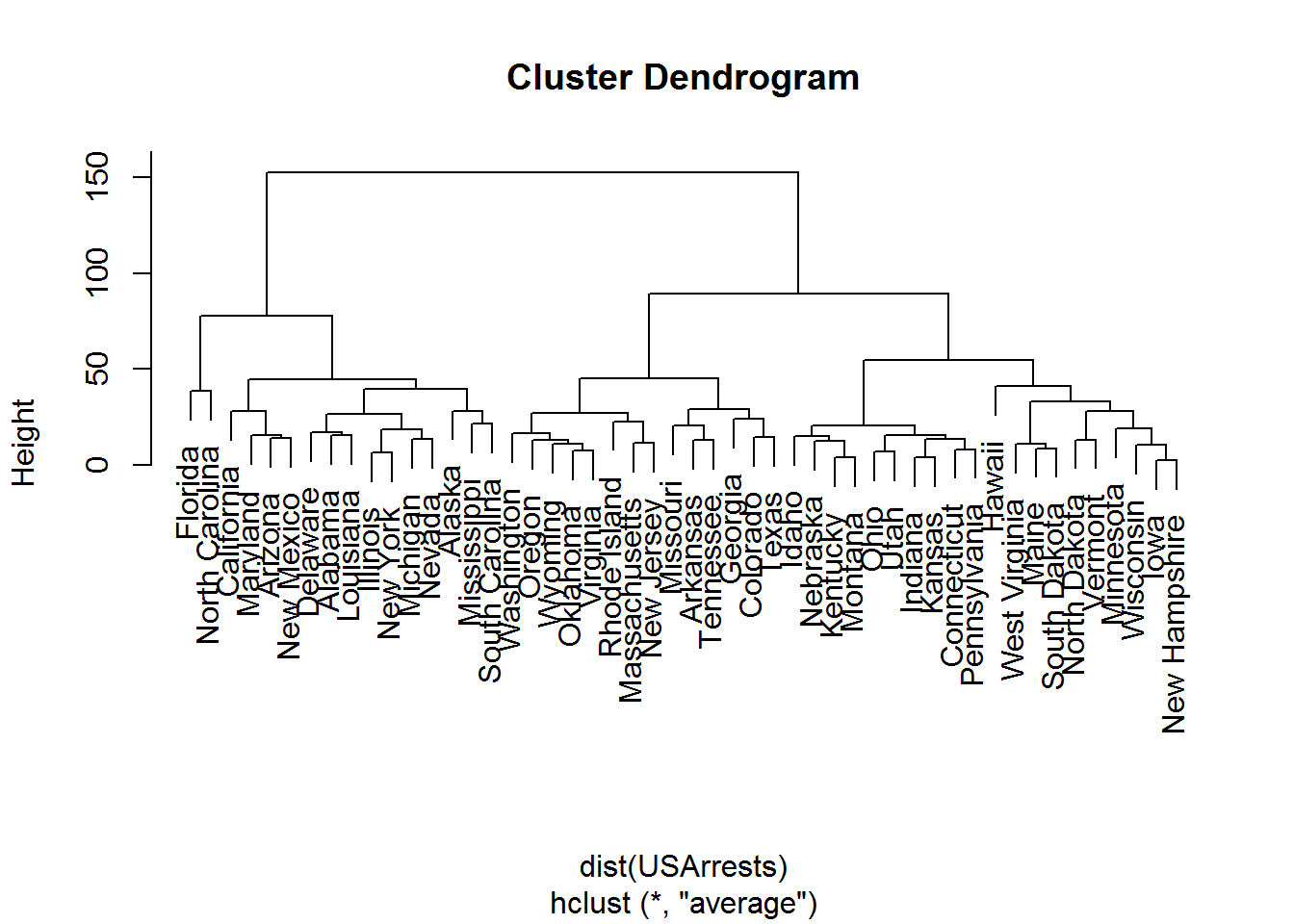啰嗦几句,可以看到聚类的结果是一个树状图,也就是说,具体聚成几类不是层次聚类决定的,基本上是需要通过一个剪枝的过程来完成,比如,想要两类就划一条水平的线,刚好和树型图相交两次,化为左右两棵树,便是需要的两类.

## 基于中心的聚类

基于中心的聚类当是一系列k-means的方,包括一些模糊的聚类:fuzzy-k-means等方法 .

基于中心的聚类是基于这样的一个假定:

每个聚类都有一个中心点,通过迭代不断使得各个点到它聚类的中心距离最短.

在这里,就可以引入聚类的一些模式化的概念,大部分聚类方法是可以看做一个贪婪算法的案例,它没有一个全局最小化的方法,而是在每一步选取一个最优解,然后再把最优解串联起来,得到最后的结果.这样的局部最优解的结合不一定是全局的最优解,这一点要牢记.

先定义一些参数用于叙述：

• (N)观测值的数量

• (K(<N))聚类的个数

• (k=C(i)) 第i个观测值属于第k个类

一个常用的损失函数是这样定义的

[W(C)=\frac{1}{2}\sum^K{k=1}\sum_{C(i)=k}\sum_{C(i’)=k}d(x_i,x_{i’})]

这个损失函数的定义思路是将每个类中两两之间的距离做和.最小化这个损失函数就意味着最小化每个类中各个观测值之间的距离(为什么有个1/2也显而易见了吧).

另外引入(T)(total point scatter) 和 (B(C)) (between point scatter)的概念:

[T=\frac{1}{2}\sum_{i=1}^{N}\sum_{i’=1}^{N}d_{ii’}=\frac{1}{2}\sum^K_{k=1}\sum_{C(i)=k} \lgroup \sum_{C(i’)=k}d(x_i,x_{i’}) + \sum_{C(i’) \neq k}d(x_i,x_{i’}) \rgroup]

或者写作:

[T=W(C)+B(C)]

对于一组数据T是不变的,所以最小化损失函数(W(C))就等价于最大化(B(C)):

[W(C)=\frac{1}{2}\sum^K_{k=1}\sum_{C(i)=k}\sum_{C(i’) \neq k}d(x_i,x_{i’})]

这也就是为什么说聚类可以看做:使每个类内的观测值尽可能的接近(最小化(W(C))),并使得类与类之间的距离尽可能的远离(B(C)),二者其实并不矛盾.

### K-means

James MacQueen,1967最早提出kmeans的算法. kmeans的思路是假设每个类都有一个中心点,而计算的目标是最小化各个数据到它所在类中心点的距离:

[W(C)=\sum^k_{i=1}\sum_{x \in S_i}||x-\mu_i||^2]

基本算法:

1. 初始化k个中心点({ m_1,…,m_K})如果已经分为几类,则计算这几类的中心点

2. 对于一个给定的中心点,计算将所有数据分类到离它最近的那个中心点: [C(i)=argmin_k||x_i-x_k||^2]

重复1和2 直到类不发生任何改变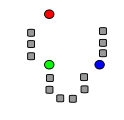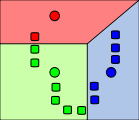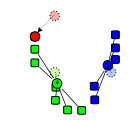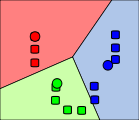kmeans的算法实际上会耗费比较多的时间,具体的复杂度为(O(n^{dk+1}\log{n}))(复杂度的部分我都未验证,来源这里),而现在常用的kmeans算法使用的是另一套较为高效的算法:Lloyds algorithm(O(n k d i)):

在给定一系列聚类初值的情况下,进行如下的步骤:

1. Assignment step: [S_i^{(t)} = \big { x_p : \big | x_p - m^{(t)}_i \big |^2 \le \big | x_p - m^{(t)}_j \big |^2 \ \forall j, 1 \le j \le k \big},] 因此(x_p)应当是归于(S_i^{(t)})类.

2. Update step:

[m^{(t+1)}_i = \frac{1}{|S^{(t)}i|} \sum{x_j \in S^{(t)}_i} x_j]

### Example

require(graphics)set.seed(123)# a 2-dimensional examplex <- rbind(matrix(rnorm(100, sd = 0.3), ncol = 2),           matrix(rnorm(100, mean = 1, sd = 0.3), ncol = 2))colnames(x) <- c("x", "y")(cl <- kmeans(x, 2))

## K-means clustering with 2 clusters of sizes 50, 50#### Cluster means:##         x       y## 1 0.92383 1.01164## 2 0.01032 0.04392#### Clustering vector:##    2 2 2 2 2 2 2 2 2 2 2 2 2 2 2 2 2 2 2 2 2 2 2 2 2 2 2 2 2 2 2 2 2 2 2##   2 2 2 2 2 2 2 2 2 2 2 2 2 2 2 1 1 1 1 1 1 1 1 1 1 1 1 1 1 1 1 1 1 1 1##   1 1 1 1 1 1 1 1 1 1 1 1 1 1 1 1 1 1 1 1 1 1 1 1 1 1 1 1 1 1#### Within cluster sum of squares by cluster:##  8.139 7.396##  (between_SS / total_SS =  74.0 %)#### Available components:####  "cluster"      "centers"      "totss"        "withinss"##  "tot.withinss" "betweenss"    "size"         "iter"##  "ifault"

library(ggplot2)cluster=as.factor(cl$cluster)qplot(x[,1],x[,2], col = cluster)+geom_point(aes(cl$centers[,1],cl$centers[,2]),size=4, col = 1:2, pch = 8, cex = 2)+ labs(x = "X", y = "Y",title="kmeans")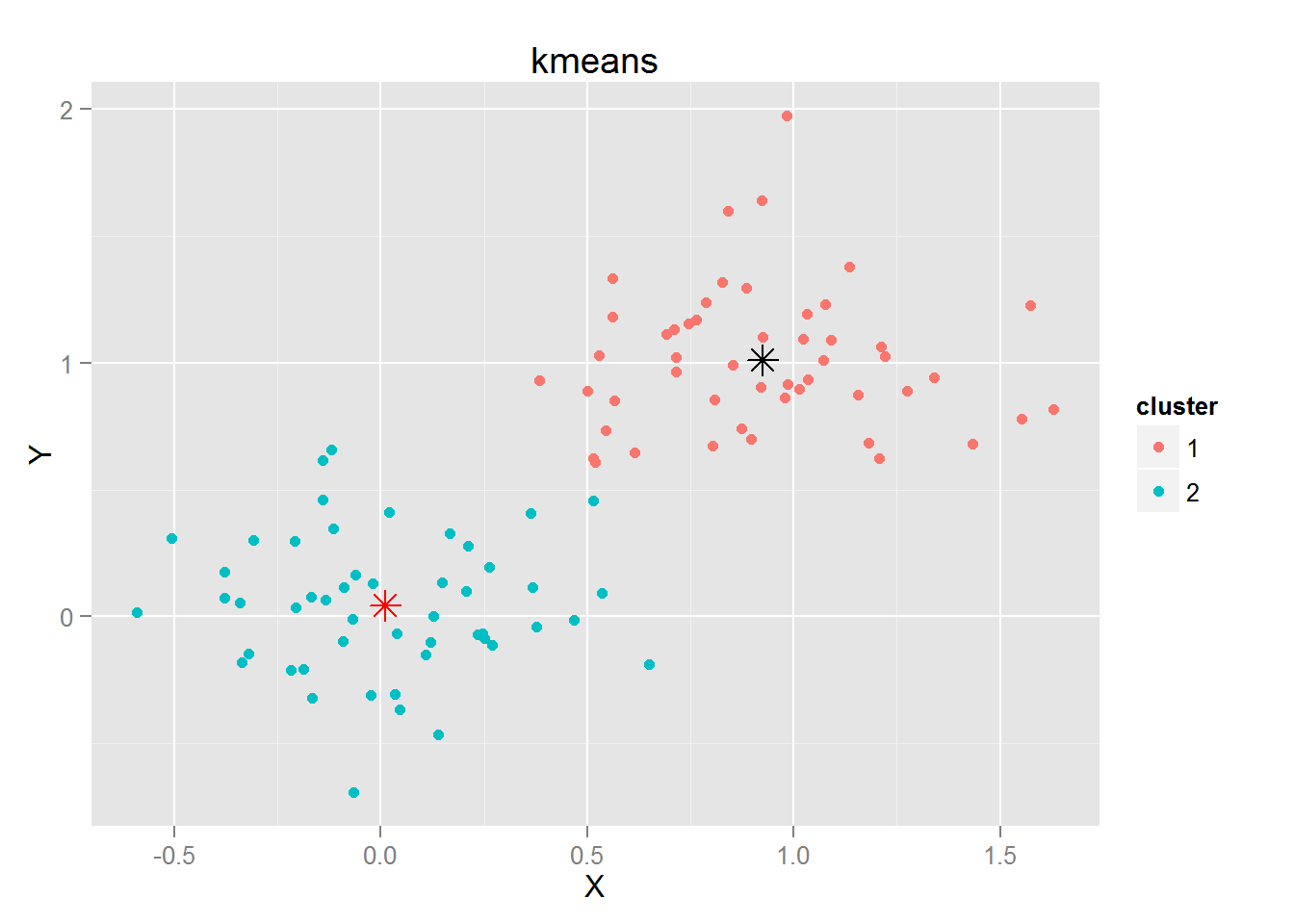## Distribution-based clustering ## 基于分布的聚类 基于分布的聚类是一套聚类的算法,假设:每个聚类来自于预先假定的分布(比如正态分布),和接下来要提到的基于密度的聚类相比,如果假设数据来源于一个高斯模型,则它的分布形式是一个具有类似球状的样子,而基于密度的聚类可以是任意的形状. 基于分布的聚类基本上最常用的就是高斯混合模型(GMM)可以参考这里wiki GMM的求解是基于EM算法完成的: 未知参数的先验分布为: $$\pi(\boldsymbol{\theta}) = \sum_{i=1}^K\phi_i \mathcal{N}(\boldsymbol{\mu_i,\Sigma_i})$$ 第i个观测值是从(\mathcal{N}(\boldsymbol{\mu_i,\Sigma_i})中依据权重 (\phi_i) 生成的(是由混合高斯分布生成的),对于某个未知参数的求解,使用EM算法. [ p(\boldsymbol{\theta | x}) = \sum_{i=1}^K\tilde{\phi_i} \mathcal{N}(\boldsymbol{\tilde{\mu_i},\tilde{\Sigma_i}})] 更新$ (, )(和)$. #### Example 恩恩,mclust包里面的clustCombi函数提供了合适的渠道来快速GMM~ 哈哈~省心.. library(mclust) ## Warning: package ‘mclust’ was built under R version 3.1.2 ## Package ‘mclust’ version 4.4## Type ‘citation("mclust")’ for citing this R package in publications. output <- clustCombi(x)output #### EM/BIC Solution## —————#### Number of components: 2## Model name: EII#### Component num.1:## proportion: 0.50## mean: 0.01 0.05## Component num.2:## proportion: 0.50## mean: 0.92 1.01#### Combining steps## —————#### Step | Classes combined at this step | Classes labels after this step## ——-|——————————-|——————————-## 0 | — | 1 2## 1 | 1 & 2 | 1#### Classification for K classes: output$classification[[K]]##  Combining matrix (K classes -> (K-1) classes): output$combiM[[K]] plot(output, x,what="classification",reg=2)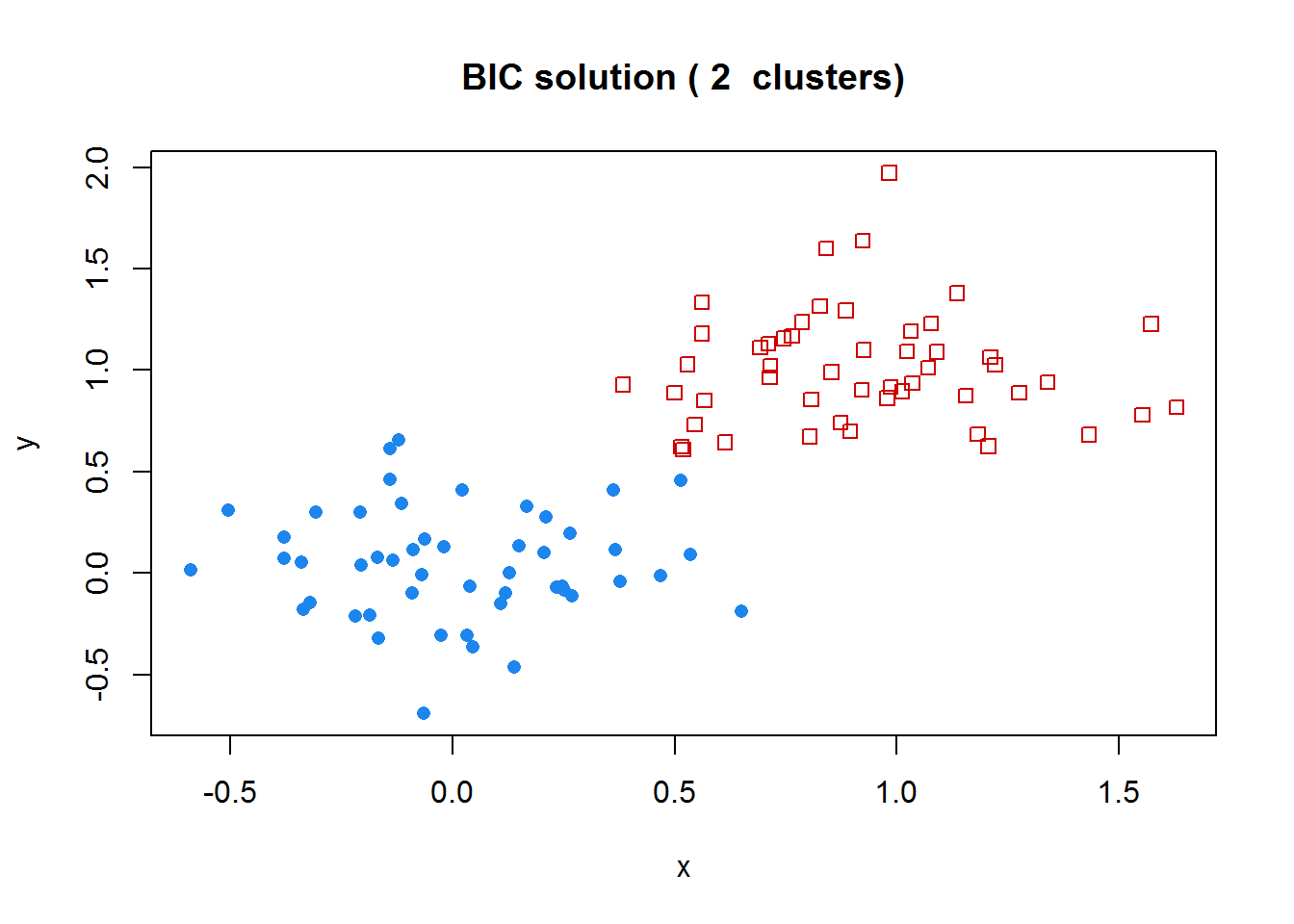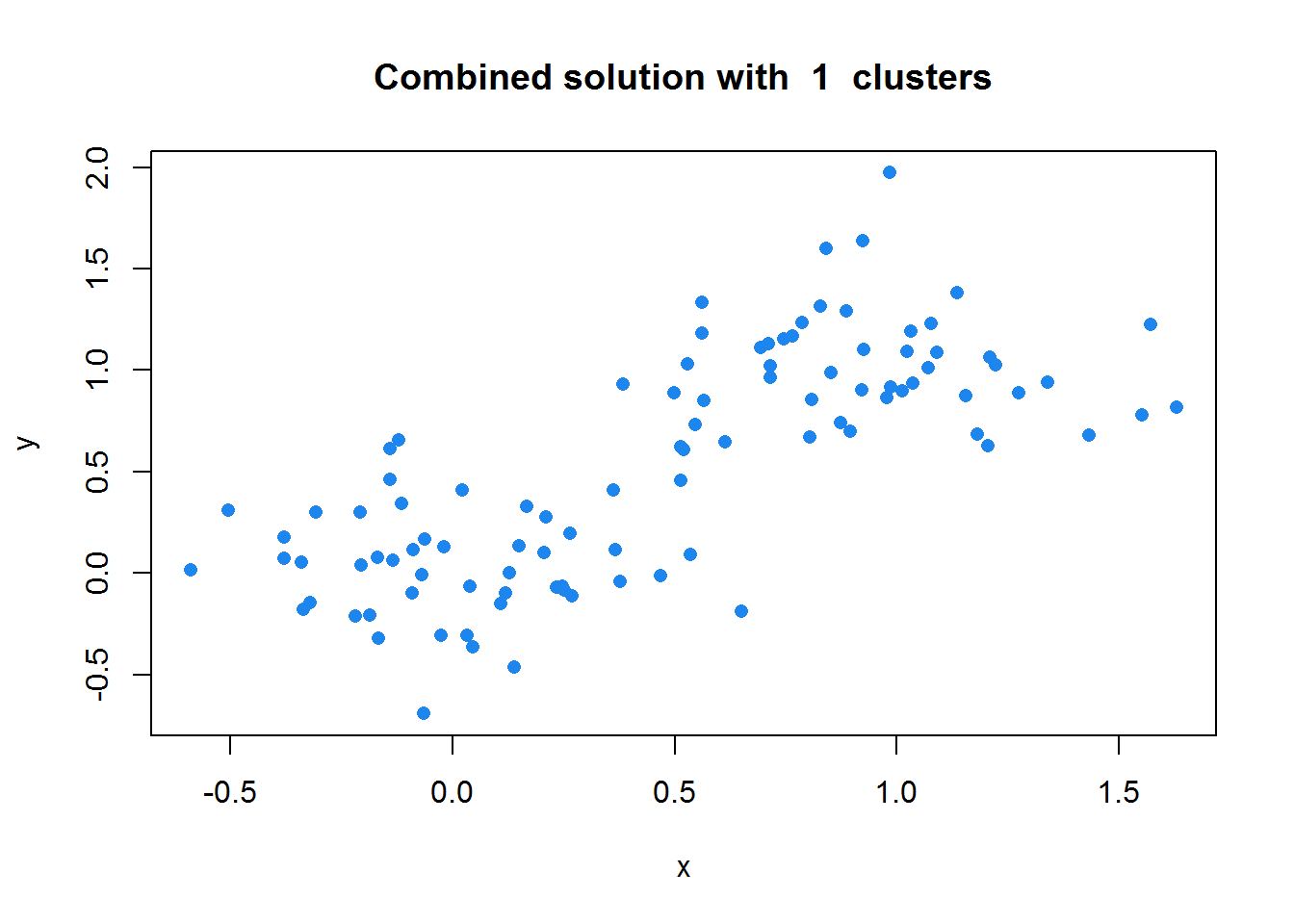## Density-based clustering ## 基于密度的聚类 基于密度的聚类是将一个类定义为:显著比其他数据有更高的密度.常用的算法有DBSCANOPTICS ### DBSCAN DBSCAN的定义是基于density reachability(密度可达性)实现的,p和q两个点称为直接密度可达是指p和q之间的距离小于一个给定的距离(\epsilon),称p,q是密度可达是指:存在一个序列 (p_1,\ldots,p_n)使得(p_1 = p)并且(p_n = q) 其中每一个 (p_{i+1})都和(p_i)相连通.思路很像基于连通的聚类是不是? 对于一个聚类,具有以下两个性质(DBSCAN的游戏规则): 1. 一个聚类内的所有点都是密度可达的. 2. 如果一个点对于聚类中每个点都是密度可达的,那这个点就是这个类中的一个. B和C是密度可达的: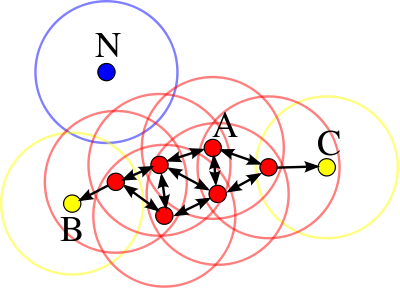DBSCAN需要两个参数来开始执行运算,一个是eps代表(\epsilon) 最短距离,MinPts代表一个聚类中最小点数.伪代码算法如下: ## pseudocodeDBSCAN(D, eps, MinPts) C = 0 for each unvisited point P in dataset D mark P as visited NeighborPts = regionQuery(P, eps) if sizeof(NeighborPts) < MinPts mark P as NOISE else C = next cluster expandCluster(P, NeighborPts, C, eps, MinPts)expandCluster(P, NeighborPts, C, eps, MinPts) add P to cluster C for each point P’ in NeighborPts if P’ is not visited mark P’ as visited NeighborPts’ = regionQuery(P’, eps) if sizeof(NeighborPts’) >= MinPts NeighborPts = NeighborPts joined with NeighborPts’ if P’ is not yet member of any cluster add P’ to cluster CregionQuery(P, eps) return all points within P’s eps-neighborhood (including P) ## Examples library(fpc) set.seed(123) n <- 600 x1 <- cbind(runif(10, 0, 10)+rnorm(n, sd=0.2), runif(10, 0, 10)+rnorm(n, sd=0.2)) ds <- dbscan(x1, 0.2)# run with showplot=1 to see how dbscan works. ds ## dbscan Pts=600 MinPts=5 eps=0.2## 0 1 2 3 4 5 6 7 8 9 10## border 16 6 9 6 3 5 4 4 6 7 7## seed 0 52 51 53 57 53 54 54 51 53 49## total 16 58 60 59 60 58 58 58 57 60 56  plot(ds, x1)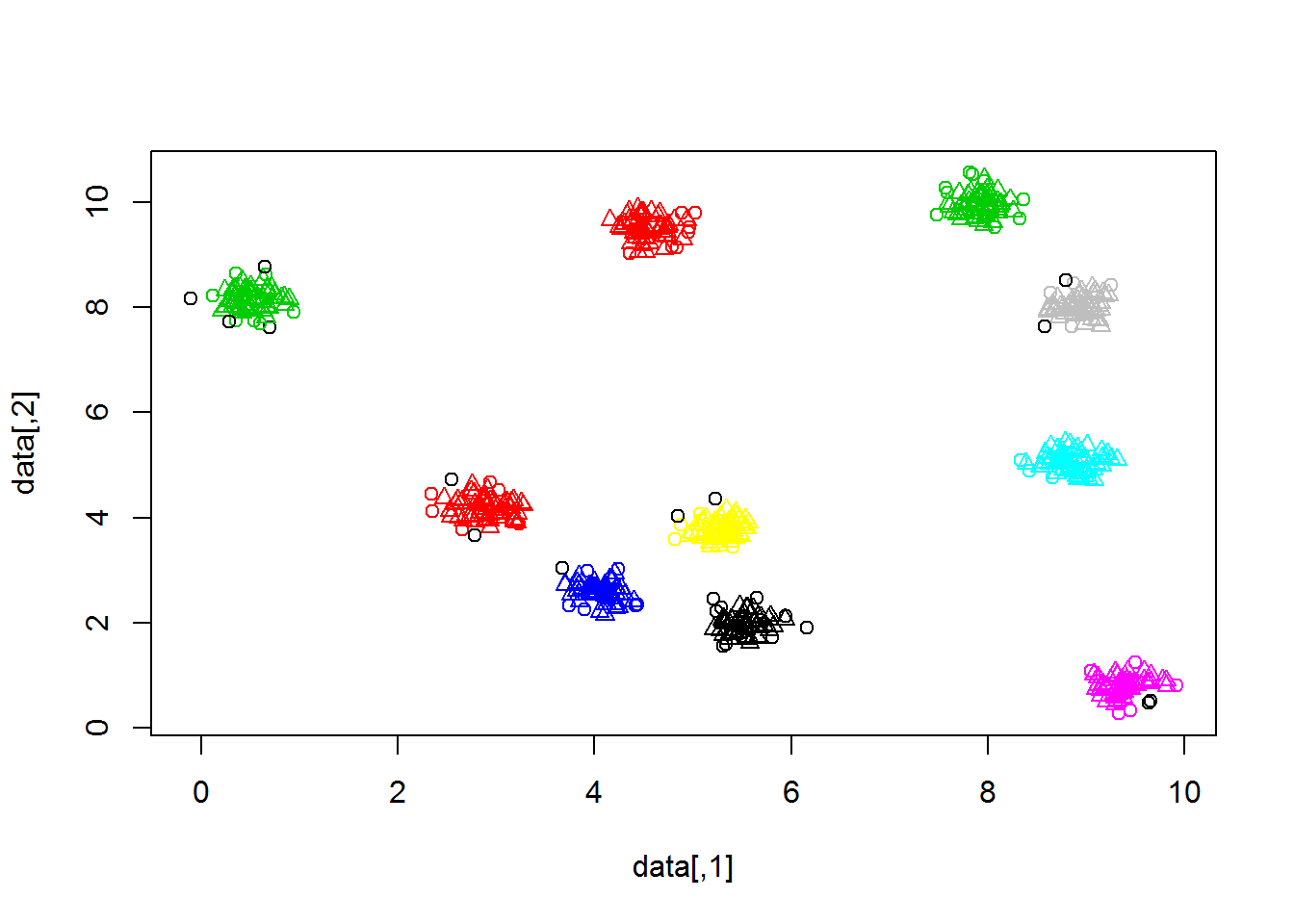### OPTICS OPTICS和DBSCAN聚类相同,都是基于密度的聚类,但是,OPTICS的好处在于可以处理不同密度的类,结果有点像基于连通性的聚类,不过还是有些区别的. 上段伪代码:  OPTICS(DB, eps, MinPts) for each point p of DB p.reachability-distance = UNDEFINED for each unprocessed point p of DB N = getNeighbors(p, eps) mark p as processed output p to the ordered list if (core-distance(p, eps, Minpts) != UNDEFINED) Seeds = empty priority queue update(N, p, Seeds, eps, Minpts) for each next q in Seeds N’ = getNeighbors(q, eps) mark q as processed output q to the ordered list if (core-distance(q, eps, Minpts) != UNDEFINED) update(N’, q, Seeds, eps, Minpts)In update(), the priority queue Seeds is updated with the \varepsilon-neighborhood of p and q, respectively: update(N, p, Seeds, eps, Minpts) coredist = core-distance(p, eps, MinPts) for each o in N if (o is not processed) new-reach-dist = max(coredist, dist(p,o)) if (o.reachability-distance == UNDEFINED) // o is not in Seeds o.reachability-distance = new-reach-dist Seeds.insert(o, new-reach-dist) else // o in Seeds, check for improvement if (new-reach-dist < o.reachability-distance) o.reachability-distance = new-reach-dist Seeds.move-up(o, new-reach-dist)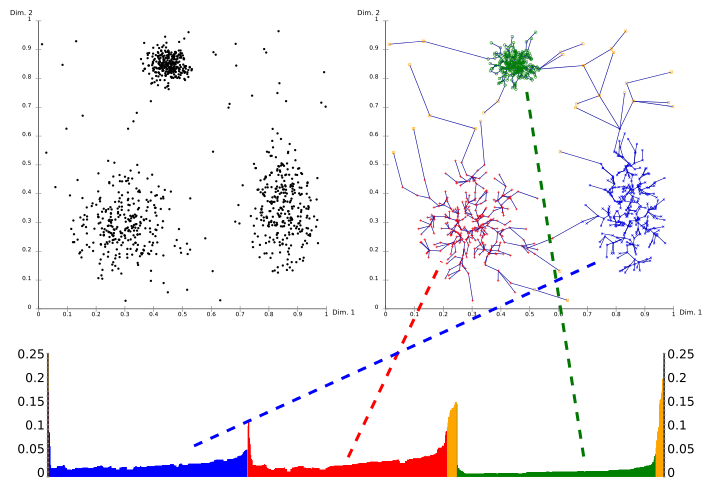## Model-based clustering ## 基于模型的聚类 基于模型的聚类,又叫做混合线性回归(我更倾向于后者)研究的问题包含了如何进行聚类和如何将聚类的结果进行回归,最简单的莫过于线性回归的结果. 基于模型的聚类假设数据来自于多个模型(线性,非线性,而且是有明确的自变量和相应变量….),类似如下的形式: 定义 1(\pi_j,j=1,2…g) 是分属不同模型的概率，对于来自于(g\ge 2) 个线性回归模型的 ({X,Y}),称具有以下性质的模型为混合回归模型(mixture regression model) (^{}) [Y=X’\beta_i+\sigma_i \epsilon_i] 模型的求解是一套最小化损失函数的思路来完成的,比如下文提到的基于OLS准则和LDA准则的最小化函数: (\tau_{ij})取值为0或1,表示第i个观测值是否来自于第j个模型( (Y=X’\beta_j+\sigma_j \epsilon_j) ),则模型的OLS参数估计可表示为： [(\beta_1,\beta_2…\beta_g)=argmin{\sum^g_{j=1}\sum^n_{i=1}(y_i\tau_{ij}-x_i\beta_j\tau_{ij})^2}] 模型的LDA参数估计可表示为： [(\beta_1,\beta_2…\beta_g)=argmin{\sum^g_{j=1}\sum^n_{i=1}|y_i\tau_{ij}-x_i\beta_j\tau_{ij}|}] 具体的求解过程是一套EM算法(EM算法也是一套不能达到极值的贪婪算法,在每一步都是取当前情况下的最优解,可能最后得到的不是全局最优而是局部最优). 比如一个稳健回归的EM算法是如下的形式: 1.选取初值: [\theta = (\beta_1,\sigma^2_1,\pi_1,…,\beta_g,\sigma^2_g,\pi_g)] 2.E-Step: 在第k+1次迭代，计算： [ \tau_{ij}= \frac{\pi_i^{(k)} \sigma_i^{-1(k)}exp(-\sqrt2 | Y_j -X_j’ \beta_i^{(k)}|/\sigma_i^{(k)})}{\sum^g_{m=1} \pi_m^{(k)} \sigma_m^{-1(k)}exp(-\sqrt2 | Y_j -X_j’ \beta_m^{(k)}|/\sigma_m^{(k)})}] [\delta_{ij}=\frac{\sigma_i^{(k)}}{\sqrt2 |Y_j-X_j’\beta_i^{(k)}|}] 3.M-Step: 使用如下数值： [\pi_i^{k+1}=\frac{1}{n}\sum_{j=1}^n\tau_{ij}^{(k)},\beta_i^{(k+1)}=\lgroup \sum_{j=1}^{n}\tau_{ij}^{(k+1)}\delta_(ij)^{(k+1)}X_jX_j’ \rgroup^{-1} \lgroup \sum_{j=1}^{n}\tau_{ij}^{(k+1)}\delta_(ij)^{(k+1)}X_jY_j’ \rgroup] [ \sigma_i^{2(k+1)}=\frac{2\sum_{j=1}^{n}\tau_{ij}^{(k+1)}\delta_(ij)^{(k+1)}(Y_j-X_j’\beta_i^{(k+1)})^2}{\sigma_j=1^n\tau_{ij}^{(k+1)}}] 最大化： [\sum_{j=1}^n\sum_{i=1}^g\tau_{ij}log\pi_i-\frac{1}{2}\sum_{j=1}^n\sum_{i=1}^g\tau_{ij}log\sigma_i^2-\sum_{j=1}^n\sum_{i=1}^g\frac{\tau_{ij}\delta_{ij}(Y_j-X_j’\beta_i)^2}{\sigma_i^2}] #### Example 看起来EM算法相对来说是一套复杂的方法,我自己做了另外一套的估计方法,效果还不错,因为涉及到自己的算法,就不再展示代码了,直观的做一个图来说明我所做的结果: 两个线性模型生成的数据,然后用一个算法估计两条直线的参数…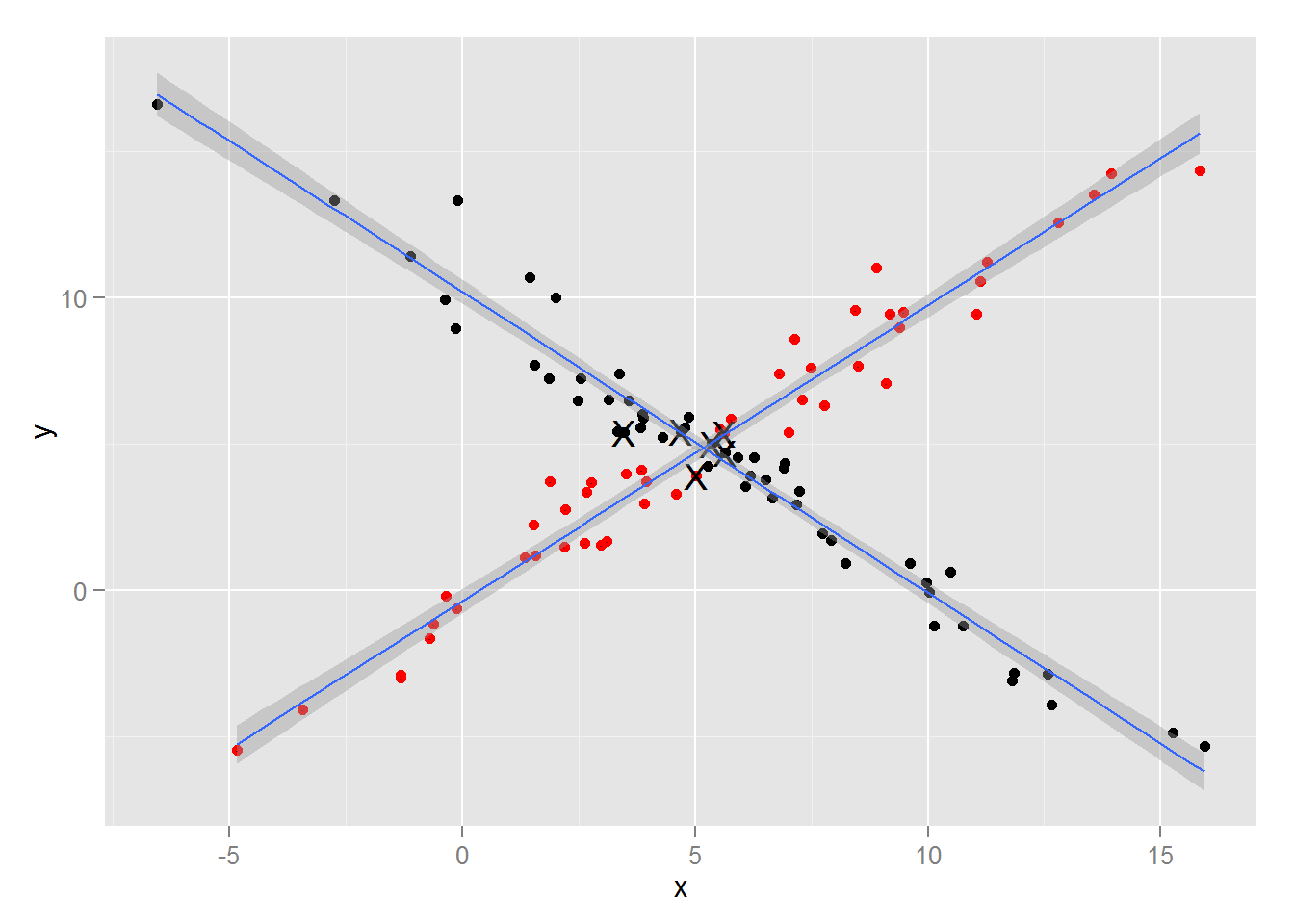## Others ## 其他聚类方法 聚类的方法远不止我提到的这几个,事实上,从最早的层次聚类开始,聚类的方法的发展就从未停止过.至今,统计学家,计算机学家已经贡献出了上百种的聚类方法,我所提到的,只是沧海一粟,不过这一粟,不过是我们常吃的五谷罢了~ 除了以上提到的聚类方法,实际上,还有很多不错的聚类框架:比如接下来要提到的谱聚类,Canopy,不过和之前方法不同的是,这两种方法并不能单独存在,而是在某些特定场合下与传统的聚类方法相结合以获得不错的结果. ### Spectral clustering ### 谱聚类 谱聚类最早来自于图像的识别领域,最早提出谱聚类的人我到现在也没有考证到,不过目测最基本的算法出来是要被吐槽的(因为实际上没太多理论价值),而在后来,谱聚类演化到图像识别领域,并且和图论的算法相结合,得到了不错的进展,反过来,在统计和机器学习等领域大放异彩,值得参考的是数据之城的博文.和A Tutorial on Spectral Clustering. 首先来概括下最直观,最简单的谱聚类:主成分+Kmeans.说完了…所以这就是为什么我觉得最早谱聚类的雏形并没有很多引用,甚至没有被提到,当然,没有被提到分两种情况,第一种是因为论文实在是太厉害,所有人都知道里面的知识,所以不用提到,比如AIC准则的论文,多少论文里面都会用AIC,看看有几个会提到1957年的那篇论文,第二种呢,是因为论文所涉及的理论和方法并不是很fancy,再后面推广什么不提原文也不会有太多心理压力,我猜谱聚类是因为后者,来看下谱聚类的算法: 1. 给出(N)个数据点两两之间的相似性。也就是一个(NN)的相似性矩阵(A)(A(i,j))代表(i)(j)两个数据点的相似度，数值越大则表示越相似。注意(A(i,j)=A(j,i)),(A(i,i)=0). 2. 计算矩阵(D)，使它的对角元是(A)矩阵的对应的那一列(或行)的值之和，其余地方为0。也就是使得 [D(i,i)=\sum_jA(i,j)] 3. (B=D-A) 4. (B)矩阵的前(k)个特征值和特征向量，将B矩阵投影到一个(k)维空间。第(i)个特征值的第j个值，就表示第j个数据点在k维空间中第i维的投影。就是说如果把k个特征向量并成一个(Nk)的矩阵，则每一行代表一个数据点在(k)维空间的坐标。 5. 根据每个数据点的(k)维空间坐标，使用K-means或者其它聚类算法在k维空间对数据进行聚类。 #### Example 对本例使用欧式距离计算相似度,然后计算D,B,计算特征值,特征向量,然后取80%的贡献率来筛选特征向量,做投影,再做Kmeans(取中心数为3),得到下面的结果: A <- as.matrix(dist(x,diag = T))D <- diag(colSums(A))B <- D-Aeig <- eigen(B)cum <- cumsum(eig$values)/sum(eig$values)k = which(cum > 0.8)final_data <- B%%eig$vectors[,1:k]cl <- kmeans(final_data, 3)cluster=as.factor(cl\$cluster)qplot(x[,1],x[,2], col = cluster)+  labs(x = "X", y = "Y",title="Spectral Clustering")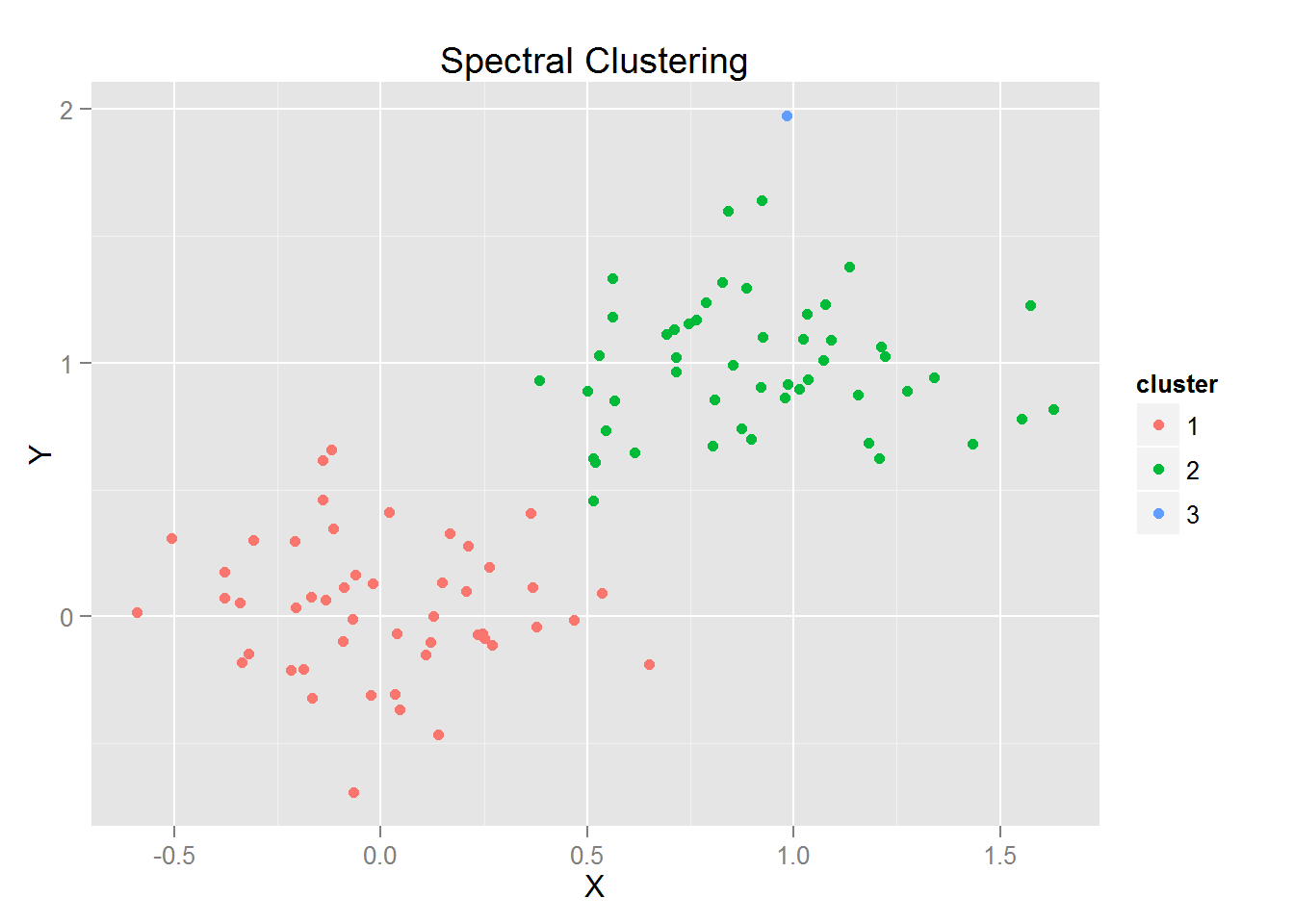调参有很多要做的事情,没办法一一列举,比如贡献率的选取,kmeans的中心数,本来 我是选择的两个中心,但是得到的根本不靠谱(毕竟数据的产生是基于kmeans的假设:有一个中心,则从一个正态分布中抽出).

另外一点要说的是谱聚类在某种意义上是增加计算复杂性的大杀器因为它是计算距离矩阵的特征值,选取其中的部分进行投影,有可能就像我之前做的一样:

• 一开始有100个数据,两个变量(x,y).

• 求完特征向量取80%再投影得到一个新的数据:100个观测值,75个变量.

• 用kmeans计算100个观测值,75变量的结果.

如果直接计算的话,kmean是处理2两个变量应该是很快的,但是在谱聚类的场合,是会做一个变量扩展,从而导致计算的复杂性增加.试想,在一个Np的问题中((N>>p))做一个谱聚类的运算代价还是比较高的.

当然谱聚类最好的方式并不是在于这个简单的算法,而是在于和图论相结合的方法,如两步法,随机游走的方法等,这些方法留到下次介绍吧~

### 变色龙聚类

别看名字如此帅气,事实上,Chameleon的名字是这样的:Chameleon:Hierarchical Clustering Using Dynamic Modeling.明尼苏达大学的三位统计学家(George Karypis,Eui-Hong (Sam) Han,Vipin Kumar)在捣鼓层次聚类的结果,不过不得不说,相对于谱聚类,展现出的效果靠谱的多.好吧,虽然说出身是层次聚类(基于连通性的聚类),但是做出的效果绝对是前沿的高端结果.

这就是论文在1999年发表出来的时候上的效果图(MD我何时才能有出头之日啊!)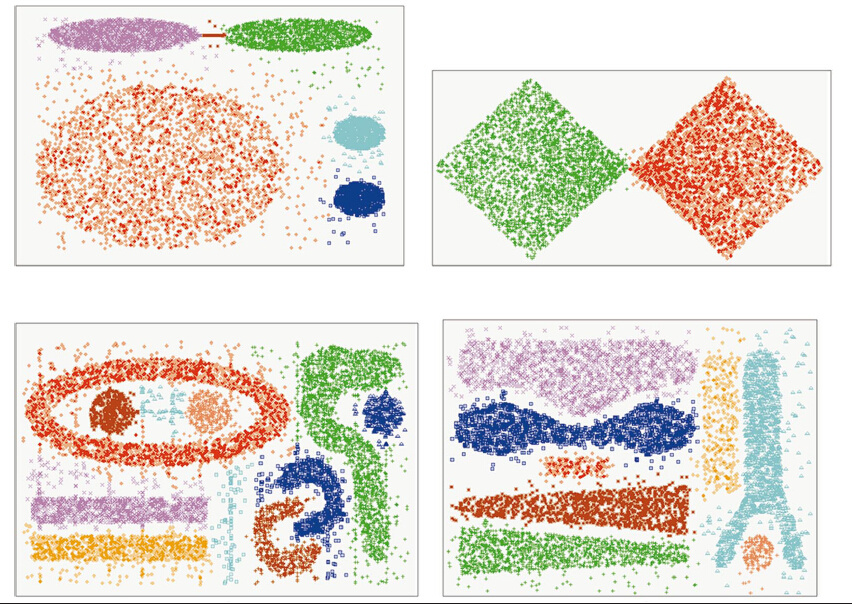说一下我对CC(Chameleon Clustering)的理解吧,CC和层次聚类类似,是一个基于连通性的聚类方法,主要的流程是:

• 先将数据聚类为一个稀疏的图(类似Knn的方法).

• 将数据分割为一个个小块.

• 再把小块根据连通性和分割平均强度来进行划分.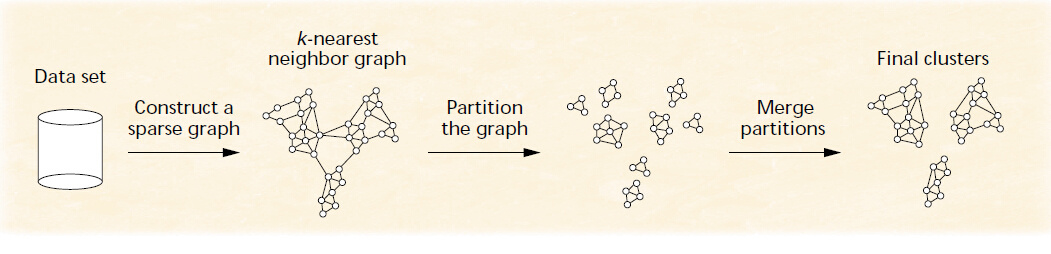### Canopy

Canopy是一个大数据下的聚类框架,最早接触canopy是在mahout的机器学习库中看到的.canopy是一个计算复杂度在(O(nk))的聚类系统,与之相对的是Canopy较低的计算精度,二者是相互取舍得到这样的结果.

Canopy来自于Andrew McCallum, Kamal Nigam and Lyle Ungar in 2000.,Canopy的意思是华盖,苍穹(苍穹聚类是不是一个很有厚重感的名字?),基本思路是:

“对于每一个传统聚类,都会存在一个Canopy使得在这个聚类内所有观测值都它里面”

“For every traditional cluster, there exists a canopysuch that all elements of the cluster are in thecanopy.”

从这里就可以看出Canopy的创造者是彻头彻尾的计算机背景(三位作者是卡内基梅隆大学Carnegie Mellon University的CS系),数学背景会选择说存在一个neighbourhood(邻域),而统计学家可能会选择说存在一个region(区间)来包含观测值,是没有Canopy这样的名字来的文艺范十足.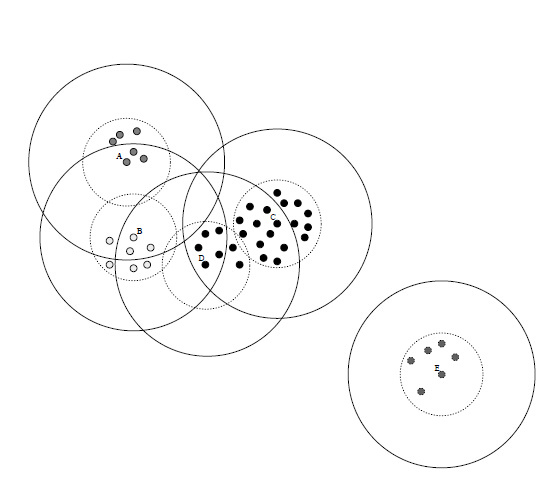另外要注意一点的是,Canopy并不一定是一个,也可以是一族,上图的虚线和实线都是代表着一个Canopy,而在他们之间,同时存在多个Cannopies,如果细分,虚线和实线的半径就是之后要定义的两个阈值,松阈值(loose distance)和紧阈值(tight distance).

#### Canopy算法

Canopy的基本算法分为两步:

1. Canopy的生成

2. 在每个Canopy下的聚类

Canopy的生成有两种模式: - 第一种,使用经验的方法来完成,比如一家医院有很多病患者的信息,根据患不同疾病的人来进行聚类(当然,有可能某个病人会患有多个疾病,这个病人会被归到多个Canopies).每类就是一个Canopy.

• 第二种,预先生成(T_1)松阈值(loose distance)和(T_2)紧阈值(tight distance).然后进行如下的计算:

1. 将需要聚类的数据转化为一个list的形式.

2. 将list中的第一个点从list中删去,转化为一个新的’canopy’的中心.

3. 对于每个list中的点,将距离在(T_1)以内的点当作这个’canopy’内的点.

4. 对于每个list中的点,将距离在(T_2)以内的点从list中删去.

5. 重复2-4步,直到list中没有任何点

6. 对于每个canopy,可以在每个canopy中应用其他代价更高的聚类,如kmeans.

和其他聚类的方法不同的是,由于存在一松一紧两个阀值,所以可能有部分,甚至是大部分观测值是落在多个canopies中的,所以得到的不是多对一对应的关系,而是多对多的关系.同时这也是canopy得到的不是clusters(聚类)而是一系列的canopy(区域).

值得吐槽的是原文说(T_1)(T_2)的生成是由用户或者是经验决定的(又来强调domian的知识)或者使用CV(cross-validation),能使用CV的话,算法该当何解?如何评判聚类的好坏呢?对于已分好类的数据问题,可以使用判断分类好坏的方法来判断(TP,TN,NT,NF),而对于纯粹的聚类问题,是没有一个确定的答案,应当使用Davies-Bouldin index或者是Dunn index来进行CV交叉验证.

### Examples

这里写一个小程序来计算各个给定数据,t1和t2的时候计算canopy的各个中心

## Calculate the center of each canopycanopy_cluster <- function(data=x,t1,t2){  n = dim(data)  data = data[sample(n),]  ## Sample the data  distance = as.matrix(dist(data,diag = T))  ## Calculate the distance matrix  judge=rep(T,n)  index=numeric(0)  for ( i in 1:n){    if(judge[i]==F) next    index=c(index,i)    pd = which(distance[i,]<t2)    judge[pd] = rep(F,length(pd))}  data[index,]}## Draw the figure of the canopycanopy_plot=function(data,t1,t2,original_data=x){  t = seq(0,2pi,length.out = 100)  n = dim(data)  t1_data = data.frame(x = 0,y = 0,num = 0)  t2_data = data.frame(x = 0,y = 0,num = 0)  for ( i in 1:n){    t1_data[((i-1)100+1):(i100),1] = data[i,1]+t1cos(t)    t1_data[((i-1)100+1):(i100),2] = data[i,2]+t1sin(t)    t1_data[((i-1)100+1):(i100),3] = rep(i,100)     t2_data[((i-1)100+1):(i100),1] = data[i,1]+t2cos(t)     t2_data[((i-1)100+1):(i100),2] = data[i,2]+t2sin(t)     t2_data[((i-1)100+1):(i*100),3] = rep(i,100)  }  p=qplot(x[,1],x[,2])+geom_point(aes(data[,1],data[,2]),size=4, col = 1:n, pch = 8, cex = 2)+  labs(x = "X", y = "Y",title="Canopy")+  geom_path(data=t1_data,aes(x=x,y=y,group=num),linetype=2)+  geom_path(data=t2_data,aes(x=x,y=y,group=num),linetype=1)return(p)}set.seed(123)data=canopy_cluster(x,t2=0.5)canopy_plot(data,t1=1,t2=0.5)